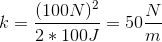# MCAT Physical : Springs and Harmonics

## Example Questions

### Example Question #11 : Rotational, Circular, And Harmonic Motion

A mass hanging in equilibrium is attached to a fixed surface on the ceiling by a spring. The mass is pulled down from the ceiling, then released and allowed to move in simple harmonic motion. The mass does not lose energy due to friction or air resistance. Which of the following would increase the frequency with which the mass moves through the equilibrium position?

Decrease displacement of the pull downward

Increase the mass

Increase stiffness of the spring

Increase displacement of the pull downward

Increase stiffness of the spring

Explanation:

This problem focuses on understanding simple harmonic motion. The formula to identify the frequency, or cycles per second, that the mass moves is given below, whereis the spring constant of the spring andis the mass of the object attached.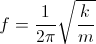Notice that the frequency does not depend on how far the mass is displaced originally. If the mass is displaced more, it will simply be moving faster when it goes through the equilibrium position. It will not, however, move through the equilibrium position any more frequently than it would if it had been displaced less.

Increasing the mass of the object attached would increase the denominator in the formula, and would therefore decrease the frequency of the harmonic motion.

Increasing the spring constant,, would increase the numerator of the formula, which would result in an increase in the frequency on the motion. The spring constant is directly proportional to the stiffness of the spring; thus, increasing stiffness will increase frequency.

### Example Question #21 : Rotational, Circular, And Harmonic Motion

A mass hanging in equilibrium is attached to a fixed surface on the ceiling by a spring. The mass pulled down from the ceiling, then released and allowed to move in simple harmonic motion. The mass does not lose energy due to friction or air resistance. At what point is the force on the mass due to the spring the greatest?

At maximum displacement from the equilibrium position

Just before it passes the equilibrium position

At the equilibrium position

At maximum displacement from the equilibrium position

Explanation:

The maximum force that the spring will exert on the mass is at maximum displacement from the equilibrium position. This can be seen with the formula for spring force.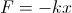is the displacement andis the spring constant. The reason that the right side of the formula is negative is because the force due to the spring is in the opposite direction of the displacement. The spring constant remains the same throughout the motion of the mass, therefore the maximum force will be generated when(displacement) is greatest.

### Example Question #22 : Rotational, Circular, And Harmonic Motion

What is the period for a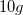block attached to a spring with a spring constant of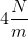after it is compressed by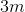and released?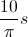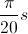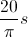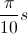Explanation:

The equation best suited to solve this question is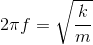, whereis the frequency of the motion,is the spring constant, andis the mass.

Two approaches can be used to solve for the period.

Since, we can first solve for the frequency, and then solve for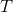.  The second method is to rearrange the equation to directly solve for: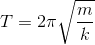. Either method can be used; it is simply a matter of preference.

Using the first method, we can plug in the given values from the question.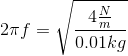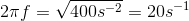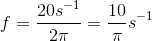Now, we need to take the reciprocal of the frequency in order to solve for the period.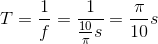Since the period is the inverse of the frequency, the answer is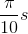.

Looking at the equation, we can see that the distance the spring is stretched or compressed has no effect on the period or frequency.

### Example Question #23 : Rotational, Circular, And Harmonic Motion

A spring is used to hang objects from the ceiling. It has a spring constant of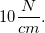The spring has a plant attached to it, and is elongated by 7cm.

What is the mass of the plant attached to the spring?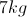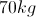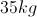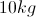Explanation:

Hooke's law is written as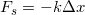, where k is the spring constant, and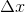is the change in position of the spring.

Since this is the upward force that will cancel out the downward force of the plant on the spring, we can set the forces equal to each other.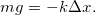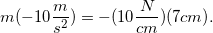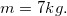### Example Question #24 : Rotational, Circular, And Harmonic Motion

A certain spring has a constant of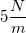.  This means that a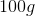mass suspended from the spring will cause which of the following results?

Shorten the spring by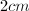Shorten the spring by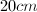Lengthen the spring byLengthen the spring by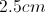Lengthen the spring byLengthen the spring byExplanation:

Hooke's law states the formula for the force of a spring: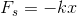The distorting force on a spring, whether lengthening or shortening, is opposed by the spring, hence the negative sign. In our question, the distorting force is caused by gravity acting on the mass, allowing us to set the force of the spring equal and opposite to the force fo gravity on the mass.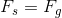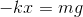Use our given values for the spring constant, mass, and acceleration of gravity to find the resultant displacement.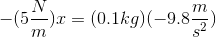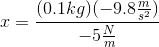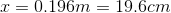Hanging the mass from the spring will cause it to stretch approximately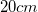.

### Example Question #25 : Rotational, Circular, And Harmonic Motion

Consider the following system: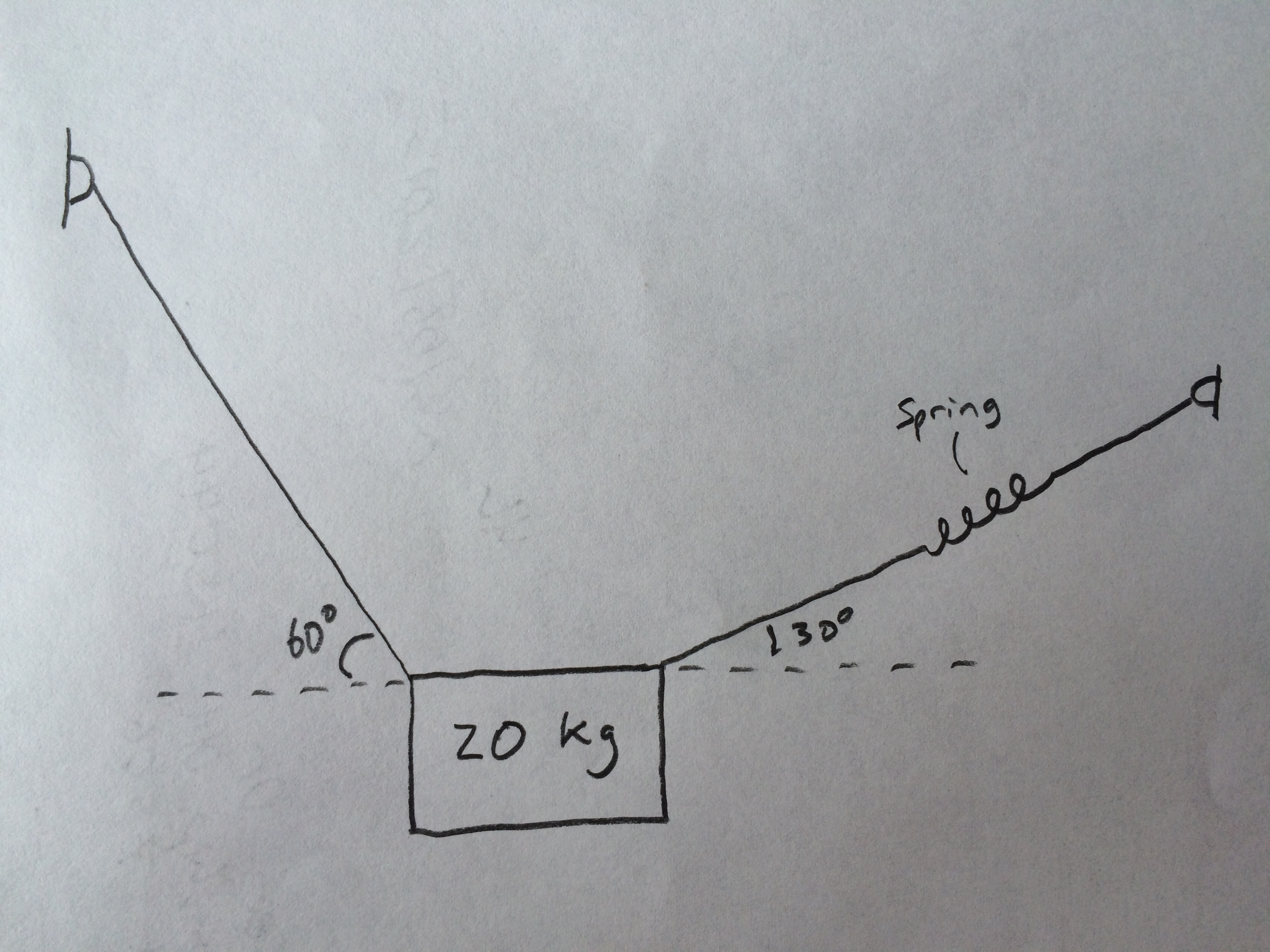What is the constant of the spring if it is storing a potential energy of 100J and the system is in equilibrium?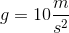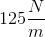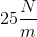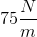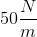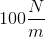Explanation:

There are a few steps to this problem. First we need to know the tension on the spring. From there we can calculate the spring constant, knowing the potential energy it is storing.

Let's draw in all of the forces to help visualize the system: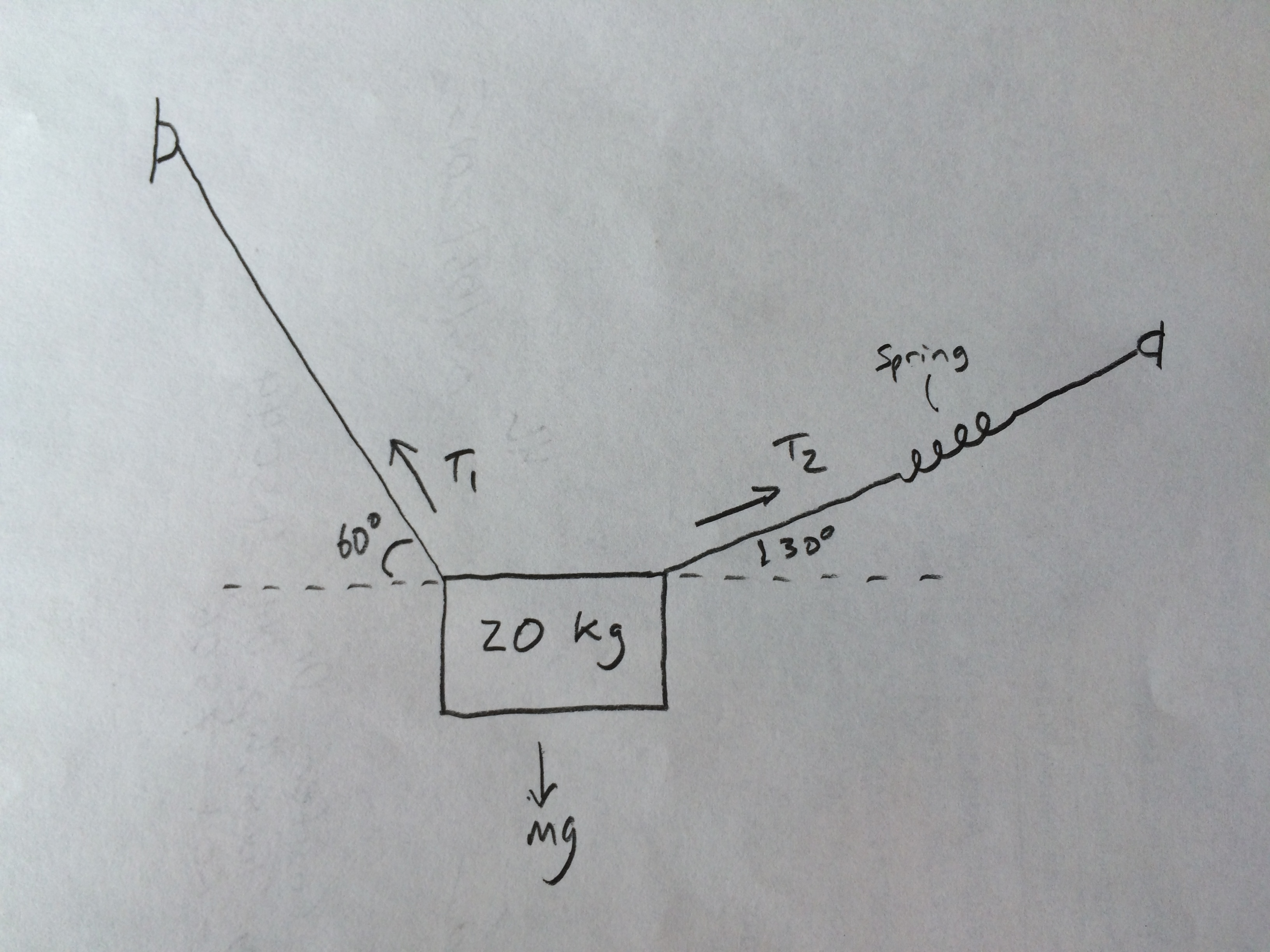If the system is at equilibrum, then all of the forces must cancel out. Separating the forces into their components we get:

x-components: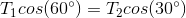y-components: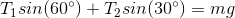We can solve these equations simultaneously for T1 and T2. Since we only need to know T2, let's rearrange the first equation for T1: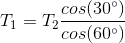Substituting this into the second equation, we get: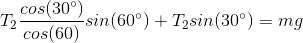Rearranging for T2: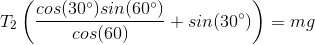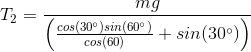Using our given values, we can solve for T2: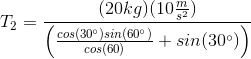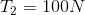This is the tension of the spring. There are two equatons that we can write out for the spring: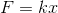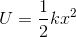The only variables we don't know areand, so we can solve these two equations simultaneously. We want, so let's rearrange the first equation for: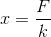Substituting this into the second equation, we get: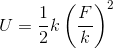Rearranging for: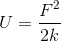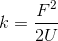We know the force and potential energy, allowing us to solve: